# R S Aggarwal Solutions for Class 11 Maths Chapter 29 Mathematical Reasoning Exercise 29B

The R S Aggarwal Class 11 solutions for the chapter Mathematical Reasoning exercise 29B is given here. This exercise is based logical connectives, compounding sentences and quantifiers and quantified statements. Here, students have to split the given sentences into simple sentences and determine whether that sentence is true or false. R S Aggarwal class 11 exercise 29B pdf contains solution of all the questions listed in this exercise.

## Download PDF of R S Aggarwal Solutions for Class 11 Maths Chapter 29 Mathematical Reasoning Exercise 29B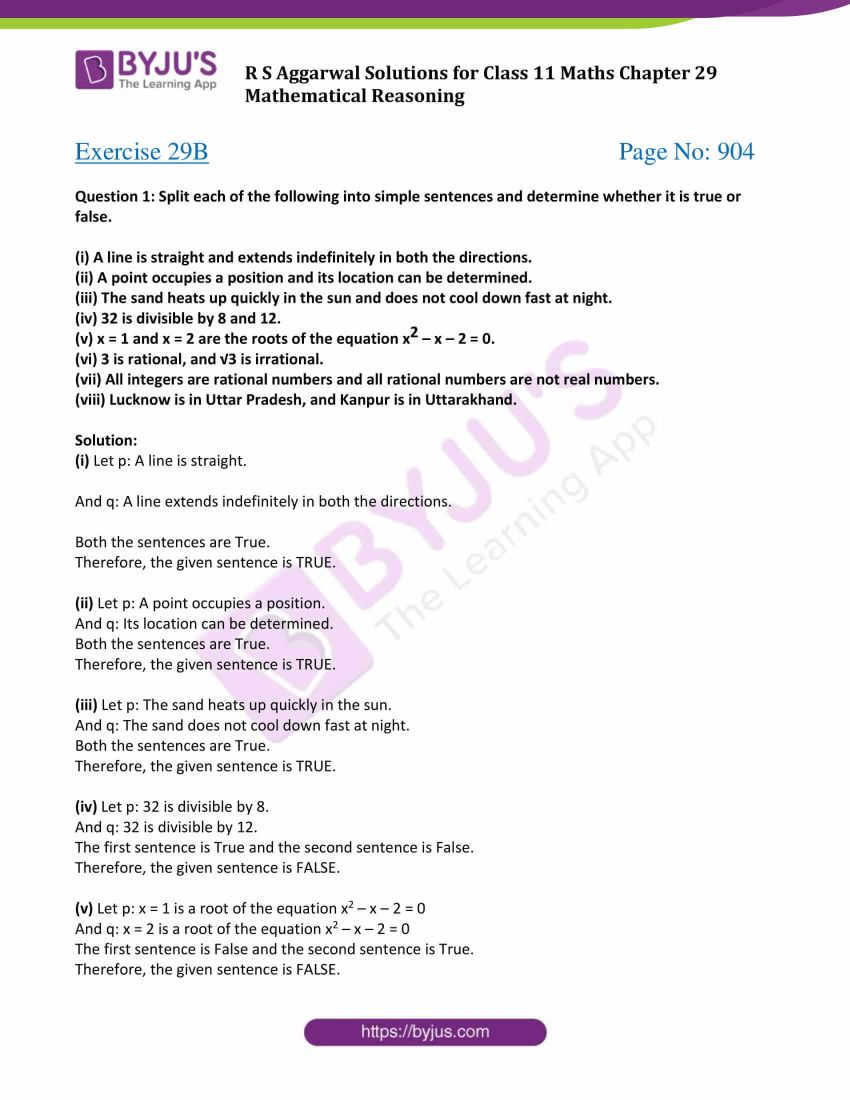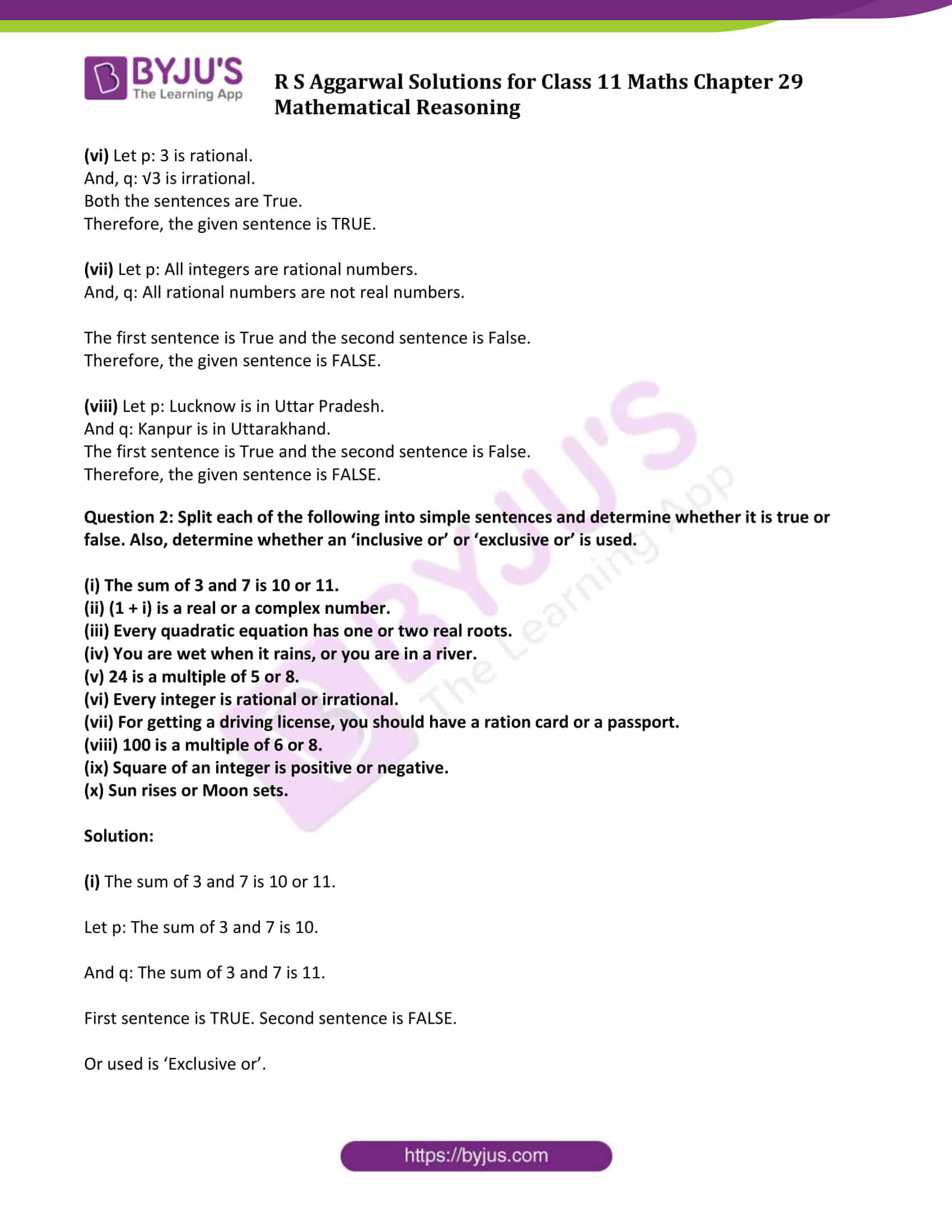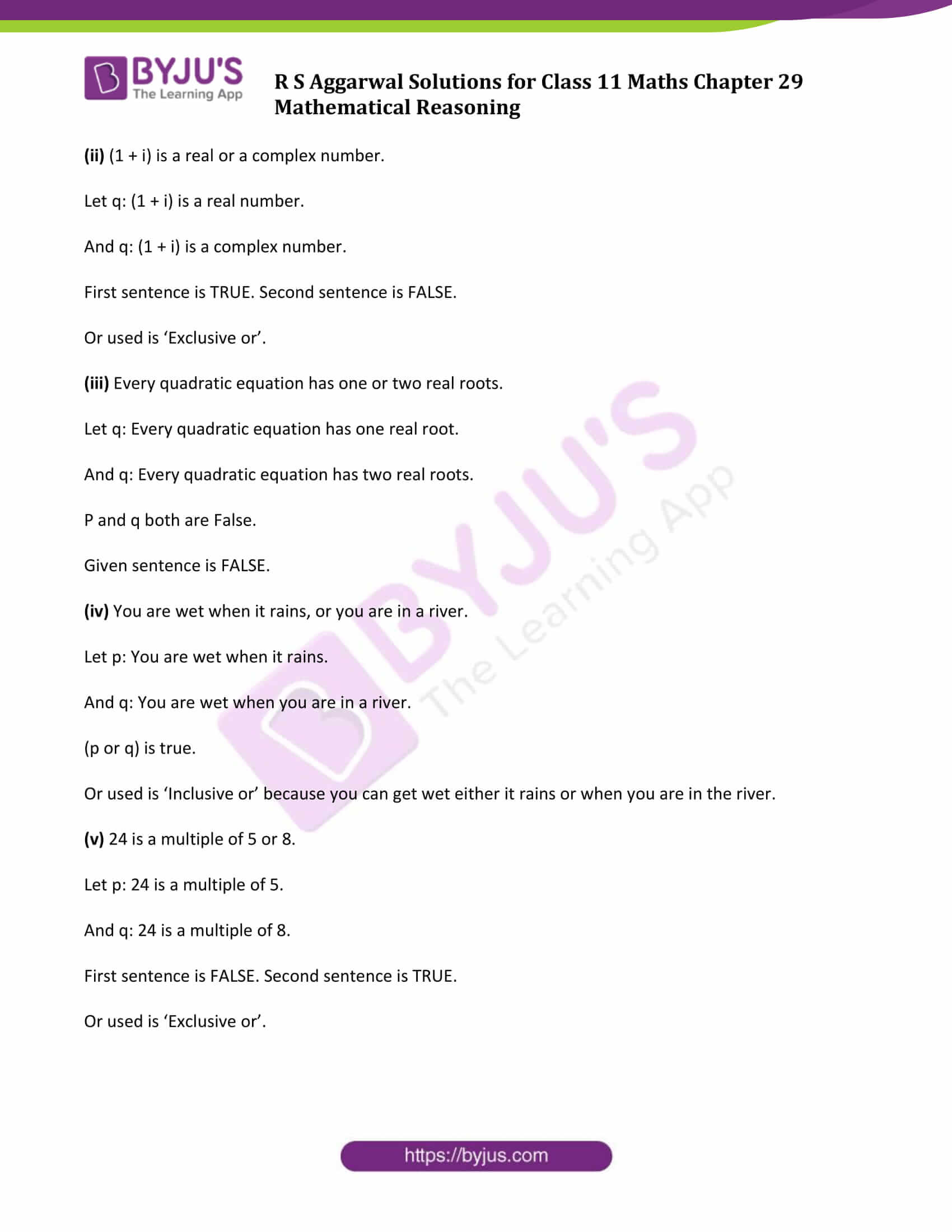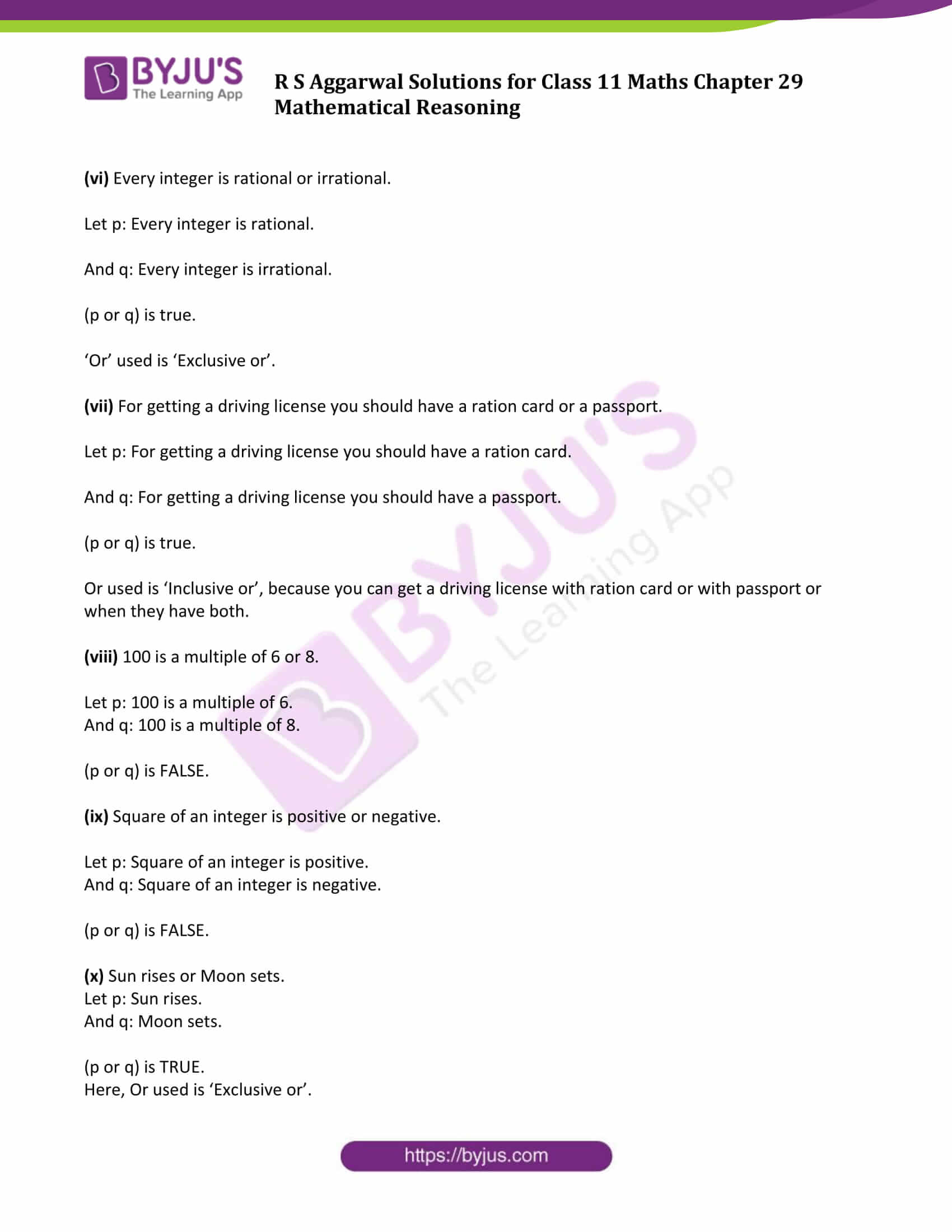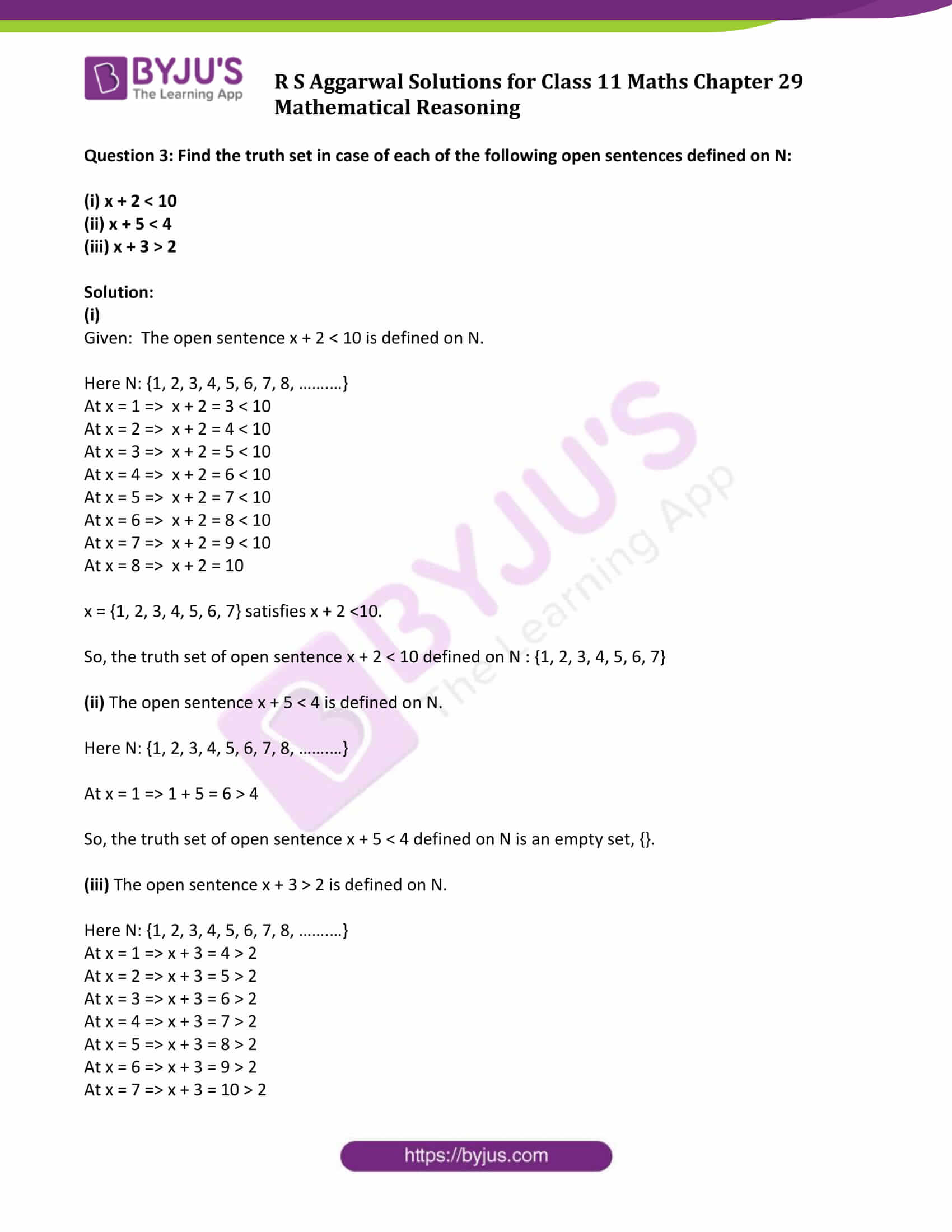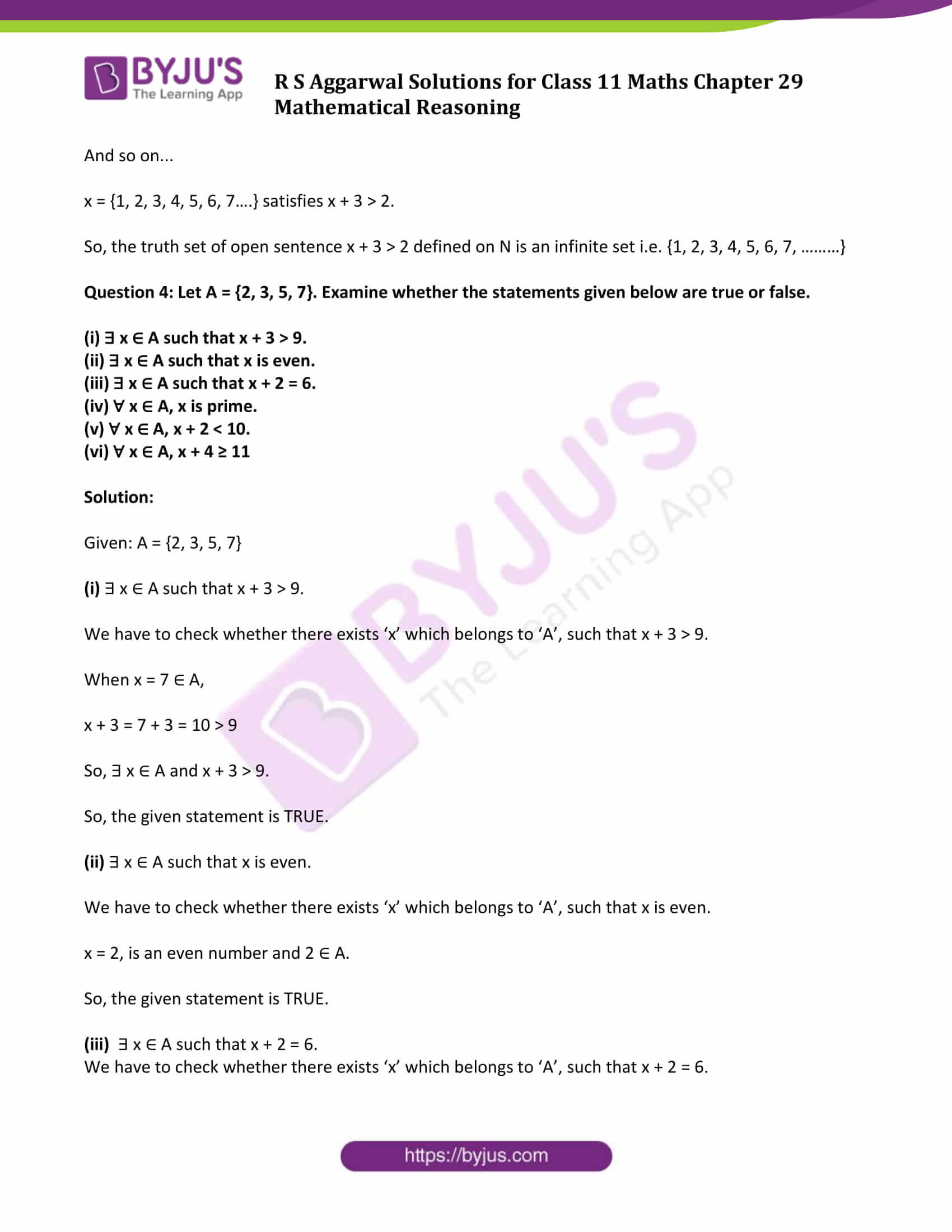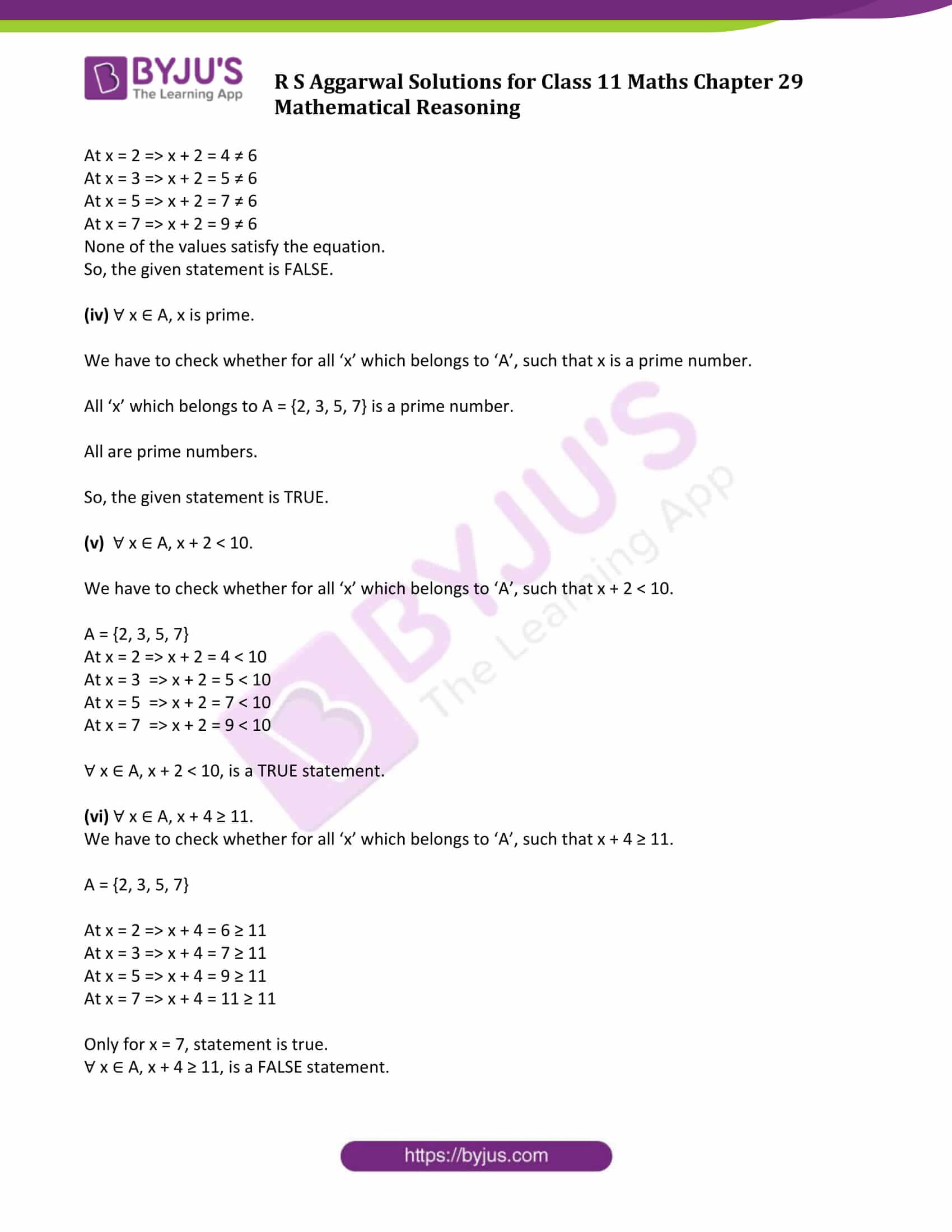### Access Answers to Maths R S Aggarwal Class 11 Chapter 29 Mathematical Reasoning Exercise 29B Page number 904

Question 1: Split each of the following into simple sentences and determine whether it is true or false.

(i) A line is straight and extends indefinitely in both the directions.

(ii) A point occupies a position and its location can be determined.

(iii) The sand heats up quickly in the sun and does not cool down fast at night.

(iv) 32 is divisible by 8 and 12.

(v) x = 1 and x = 2 are the roots of the equation x2 – x – 2 = 0.

(vi) 3 is rational, and √3 is irrational.

(vii) All integers are rational numbers and all rational numbers are not real numbers.

(viii) Lucknow is in Uttar Pradesh, and Kanpur is in Uttarakhand.

Solution:

(i) Let p: A line is straight.

And q: A line extends indefinitely in both the directions.

Both the sentences are True.

Therefore, the given sentence is TRUE.

(ii) Let p: A point occupies a position.

And q: Its location can be determined.

Both the sentences are True.

Therefore, the given sentence is TRUE.

(iii) Let p: The sand heats up quickly in the sun.

And q: The sand does not cool down fast at night.

Both the sentences are True.

Therefore, the given sentence is TRUE.

(iv) Let p: 32 is divisible by 8.

And q: 32 is divisible by 12.

The first sentence is True and the second sentence is False.

Therefore, the given sentence is FALSE.

(v) Let p: x = 1 is a root of the equation x2 – x – 2 = 0

And q: x = 2 is a root of the equation x2 – x – 2 = 0

The first sentence is False and the second sentence is True.

Therefore, the given sentence is FALSE.

(vi) Let p: 3 is rational.

And, q: √3 is irrational.

Both the sentences are True.

Therefore, the given sentence is TRUE.

(vii) Let p: All integers are rational numbers.

And, q: All rational numbers are not real numbers.

The first sentence is True and the second sentence is False.

Therefore, the given sentence is FALSE.

(viii) Let p: Lucknow is in Uttar Pradesh.

And q: Kanpur is in Uttarakhand.

The first sentence is True and the second sentence is False.

Therefore, the given sentence is FALSE.

Question 2: Split each of the following into simple sentences and determine whether it is true or false. Also, determine whether an ‘inclusive or’ or ‘exclusive or’ is used.

(i) The sum of 3 and 7 is 10 or 11.

(ii) (1 + i) is a real or a complex number.

(iii) Every quadratic equation has one or two real roots.

(iv) You are wet when it rains, or you are in a river.

(v) 24 is a multiple of 5 or 8.

(vi) Every integer is rational or irrational.

(vii) For getting a driving license, you should have a ration card or a passport.

(viii) 100 is a multiple of 6 or 8.

(ix) Square of an integer is positive or negative.

(x) Sun rises or Moon sets.

Solution:

(i) The sum of 3 and 7 is 10 or 11.

Let p: The sum of 3 and 7 is 10.

And q: The sum of 3 and 7 is 11.

First sentence is TRUE. Second sentence is FALSE.

Or used is ‘Exclusive or’.

(ii) (1 + i) is a real or a complex number.

Let q: (1 + i) is a real number.

And q: (1 + i) is a complex number.

First sentence is TRUE. Second sentence is FALSE.

Or used is ‘Exclusive or’.

(iii) Every quadratic equation has one or two real roots.

Let q: Every quadratic equation has one real root.

And q: Every quadratic equation has two real roots.

P and q both are False.

Given sentence is FALSE.

(iv) You are wet when it rains, or you are in a river.

Let p: You are wet when it rains.

And q: You are wet when you are in a river.

(p or q) is true.

Or used is ‘Inclusive or’ because you can get wet either it rains or when you are in the river.

(v) 24 is a multiple of 5 or 8.

Let p: 24 is a multiple of 5.

And q: 24 is a multiple of 8.

First sentence is FALSE. Second sentence is TRUE.

Or used is ‘Exclusive or’.

(vi) Every integer is rational or irrational.

Let p: Every integer is rational.

And q: Every integer is irrational.

(p or q) is true.

‘Or’ used is ‘Exclusive or’.

(vii) For getting a driving license you should have a ration card or a passport.

Let p: For getting a driving license you should have a ration card.

And q: For getting a driving license you should have a passport.

(p or q) is true.

Or used is ‘Inclusive or’, because you can get a driving license with ration card or with passport or when they have both.

(viii) 100 is a multiple of 6 or 8.

Let p: 100 is a multiple of 6.

And q: 100 is a multiple of 8.

(p or q) is FALSE.

(ix) Square of an integer is positive or negative.

Let p: Square of an integer is positive.

And q: Square of an integer is negative.

(p or q) is FALSE.

(x) Sun rises or Moon sets.

Let p: Sun rises.

And q: Moon sets.

(p or q) is TRUE.

Here, Or used is ‘Exclusive or’.

Question 3: Find the truth set in case of each of the following open sentences defined on N:

(i) x + 2 < 10

(ii) x + 5 < 4

(iii) x + 3 > 2

Solution:

(i)

Given: The open sentence x + 2 < 10 is defined on N.

Here N: {1, 2, 3, 4, 5, 6, 7, 8, …….…}

At x = 1 ⇒ x + 2 = 3 < 10

At x = 2 ⇒ x + 2 = 4 < 10

At x = 3 ⇒ x + 2 = 5 < 10

At x = 4 ⇒ x + 2 = 6 < 10

At x = 5 ⇒ x + 2 = 7 < 10

At x = 6 ⇒ x + 2 = 8 < 10

At x = 7 ⇒ x + 2 = 9 < 10

At x = 8 ⇒ x + 2 = 10

x = {1, 2, 3, 4, 5, 6, 7} satisfies x + 2 <10.

So, the truth set of open sentence x + 2 < 10 defined on N : {1, 2, 3, 4, 5, 6, 7}

(ii) The open sentence x + 5 < 4 is defined on N.

Here N: {1, 2, 3, 4, 5, 6, 7, 8, …….…}

At x = 1 ⇒ 1 + 5 = 6 > 4

So, the truth set of open sentence x + 5 < 4 defined on N is an empty set, {}.

(iii) The open sentence x + 3 > 2 is defined on N.

Here N: {1, 2, 3, 4, 5, 6, 7, 8, …….…}

At x = 1 ⇒ x + 3 = 4 > 2

At x = 2 ⇒ x + 3 = 5 > 2

At x = 3 ⇒ x + 3 = 6 > 2

At x = 4 ⇒ x + 3 = 7 > 2

At x = 5 ⇒ x + 3 = 8 > 2

At x = 6 ⇒ x + 3 = 9 > 2

At x = 7 ⇒ x + 3 = 10 > 2

And so on…

x = {1, 2, 3, 4, 5, 6, 7….} satisfies x + 3 > 2.

So, the truth set of open sentence x + 3 > 2 defined on N is an infinite set i.e. {1, 2, 3, 4, 5, 6, 7, ………}

Question 4: Let A = {2, 3, 5, 7}. Examine whether the statements given below are true or false.

(i) ∃ x ∈ A such that x + 3 > 9.

(ii) ∃ x ∈ A such that x is even.

(iii) ∃ x ∈ A such that x + 2 = 6.

(iv) ∀ x ∈ A, x is prime.

(v) ∀ x ∈ A, x + 2 < 10.

(vi) ∀ x ∈ A, x + 4 ≥ 11

Solution:

Given: A = {2, 3, 5, 7}

(i) ∃ x ∈ A such that x + 3 > 9.

We have to check whether there exists ‘x’ which belongs to ‘A’, such that x + 3 > 9.

When x = 7 ∈ A,

x + 3 = 7 + 3 = 10 > 9

So, ∃ x ∈ A and x + 3 > 9.

So, the given statement is TRUE.

(ii) ∃ x ∈ A such that x is even.

We have to check whether there exists ‘x’ which belongs to ‘A’, such that x is even.

x = 2, is an even number and 2 ∈ A.

So, the given statement is TRUE.

(iii) ∃ x ∈ A such that x + 2 = 6.

We have to check whether there exists ‘x’ which belongs to ‘A’, such that x + 2 = 6.

At x = 2 ⇒ x + 2 = 4 ≠ 6

At x = 3 ⇒ x + 2 = 5 ≠ 6

At x = 5 ⇒ x + 2 = 7 ≠ 6

At x = 7 ⇒ x + 2 = 9 ≠ 6

None of the values satisfy the equation.

So, the given statement is FALSE.

(iv) ∀ x ∈ A, x is prime.

We have to check whether for all ‘x’ which belongs to ‘A’, such that x is a prime number.

All ‘x’ which belongs to A = {2, 3, 5, 7} is a prime number.

All are prime numbers.

So, the given statement is TRUE.

(v) ∀ x ∈ A, x + 2 < 10.

We have to check whether for all ‘x’ which belongs to ‘A’, such that x + 2 < 10.

A = {2, 3, 5, 7}

At x = 2 ⇒ x + 2 = 4 < 10

At x = 3 ⇒ x + 2 = 5 < 10

At x = 5 ⇒ x + 2 = 7 < 10

At x = 7 ⇒ x + 2 = 9 < 10

∀ x ∈ A, x + 2 < 10, is a TRUE statement.

(vi) ∀ x ∈ A, x + 4 ≥ 11.

We have to check whether for all ‘x’ which belongs to ‘A’, such that x + 4 ≥ 11.

A = {2, 3, 5, 7}

At x = 2 ⇒ x + 4 = 6 ≥ 11

At x = 3 ⇒ x + 4 = 7 ≥ 11

At x = 5 ⇒ x + 4 = 9 ≥ 11

At x = 7 ⇒ x + 4 = 11 ≥ 11

Only for x = 7, statement is true.

∀ x ∈ A, x + 4 ≥ 11, is a FALSE statement.

## Access other exercise solutions of Class 11 Maths Chapter 29 Mathematical Reasoning

Exercise 29A Solutions

Exercise 29C Solutions

Exercise 29D Solutions

## R S Aggarwal Solutions for Class 11 Maths Chapter 29 Exercise 29B

Class 11 Maths Chapter 29 Mathematical Reasoning Exercise 29B is based on the following topics:

• Logical Connectives
• Simple statements
• Compound statements
• Logical connectives
• Compounding of sentences
• Conjunction
• Disjunction
• Truth tables
• Open sentences and their truth sets
• Quantifiers and Quantified statements